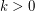Importance: Medium ✭✭
 Author(s): Bentz, Cedric
 Subject: Graph Theory
 Keywords: planar graph polynomial algorithm
 Posted by: jcmeyer on: April 18th, 2010
Conjecture   It has been shown that a-outerplanar embedding for whichis minimal can be found in polynomial time. Does a similar result hold for-edge-outerplanar graphs?

A-outerplanar graph [Baker] withis a planar graph having an embedding with at mostlayers of vertices such that after removing iteratively the vertices (and their adjacent edges) lying on the outer facetimes, we obtain the empty graph.

A-edge-outerplanar graph [Bentz] is defined to be a planar graph having an embedding with at mostlayers of edges such that after removing iteratively the edges lying on the outer facetimes, we obtain a graph with no edge. All-edge-outerplanar graphs are-outerplanar graphs.

Given a planar graph, Bienstock and Monma have shown that a-outerplanar embedding for whichis minimal can be found in polynomial time. Does a similar result hold for-edge-outerplanar graphs?

## Bibliography

C. Bentz, “Disjoint paths in sparse graphs,” Discrete Appl. Math., vol. 157, no. 17, pp. 3558–3568, 2009

D. Bienstock and C. L. Monma, “On the complexity of embedding planar graphs to minimize certain distance measures,” Algorithmica, vol. 5, no. 1–4, pp. 93–109, 1990

B. S. Baker, “Approximation algorithms for np-complete problems on planar graphs,” J. ACM, vol. 41, no. 1, pp. 153–180, 1994

* indicates original appearance(s) of problem.